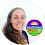## Pages

### Ideas for Teaching Number SenseIn 2013, NPR published "Scientists Put a 'Sixth Sense' For Numbers on Brain Map". The article summarized scientists' findings on a region of the brain responsible for our ability to estimate quantities.

Quantities of pencils on the floor, quantities of tiles on the ceiling, quantities of flowers in a vase. Without counting, how close can you get to estimating the correct number of objects you see?

Leading the study was Ben Harvey who was quoted as saying, "The better you are at number sensing, the better you seem to do on standardized mathematical tests."

As Math Teachers, we know how hard it is to teach number sense. It's one of those things we weave in daily as we teach more concrete topics.

We also know how difficult standardized tests can be!

Looking at number sense through the lens of this study makes it seem as innate as hearing, smelling or seeing. So is it even possible to teach number sense? From a Growth Mindset point of view, of course it is! We can ALWAYS get better at math if we work at it.

My husband and I don't care what career our daughter eventually chooses. Our #1 goal is for her to be happy. From when she enters school to the day she graduates, a big part of that happiness will be centered around her feelings about school. And those feelings, tragically, will have to do a lot with how she does on tests. So we have started doing what we can to build her number sense.

To get to know even and odd numbers, I cut out pieces of paper into even and odd shapes. She got it right away. Odd + odd = even, even + even = even, odd + even = odd, odd + odd + even = even. Later when the shapes weren't in front of us she could visualize that an odd + odd = even. Why is this important? Later when she starts adding larger numbers, it'll be easy to miscount by 1 on either side. Knowing that an even + even = even will allow her to analyze her answers.

I made this even and odd reference if you'd like something printable. It's free here in my dropbox. It doesn't have to be displayed; the pieces can be used as manipulatives in an even odd investigation

I made these place value flip books for her to help build her sense of how multi-digit numbers work.

The flip books were made to work with each other when added and subtracted. I added in adding and subtracting mats to help her see the relationship between 5432, 4321 and 9753.

I also made a set of decimal place value flip books

Dividing fractions is such a weird concept, even for us adults. I wrote this post on fraction division (with videos) to take some of the weirdness out of it.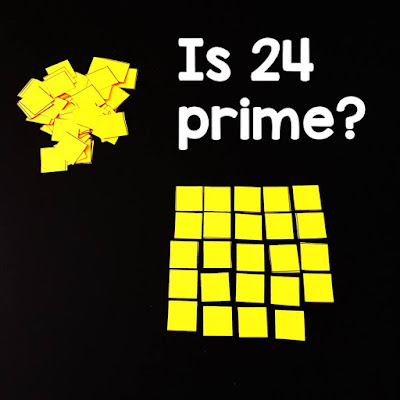Prime number investigation activity

What makes a prime number prime? One idea for introducing prime vs. composite numbers is this prime number investigation activity. Students try to arrange given numbers of paper squares into rectangles. If they can make a rectangle, their number is composite. If not, it's prime.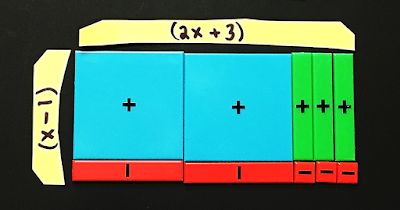Using algebra tiles to multiply polynomials

Algebra tiles are great for building all sorts of skills like multiplying polynomials, solving equations and factoring quadratics. There is a free paper set of algebra tiles linked here. To make them thicker and easier to handle, you can laminate then cut the algebra tile printable.

I loved this idea from Karyn's Blog about equivalent fractions on a multiplication chart:

When students struggle with multiplication they can end up feeling really bad about themselves! I see this a lot in Algebra 2 when we factor quadratics. Students who don't have their times tables memorized think they "can't" factor, when really it's not at all an algebra issue but is instead a math facts issue.multiplication chart with half of the products removed

This multiplication chart may be a little easier for kids to read. It takes out the duplicate products, highlights the squares and uses clear rows and columns. You can find the pdf free in my dropbox here.GCF, LCM, Prime Numbers Math Pennnt

Numbers are building blocks. (2)(2)(3) = 12 like H+H+O = water. We can break down numbers into their "prime fingerprints" just like compounds can be broken down into their elements. This prime and composite numbers pennant asks student to break down composite numbers into their prime factors, find the GCF of two composite numbers less than 100 and find the LCM of two composite numbers less than 12.GCF and LCM math word wall reference

GCF and especially LCM are weird concepts for kids. Have you seen this cake method for finding them? I love it. It makes LCM so easy! You can get this word wall reference for GCF and LCM free in my free resource libraryprime factoring math word wall reference

I recently completed a 4th grade math word wall and in it included this set of visual references for prime factoring. A few teachers commented that these references would also be helpful for older kids, so I added them to my dropbox. These prime factoring references are part of my free resource library.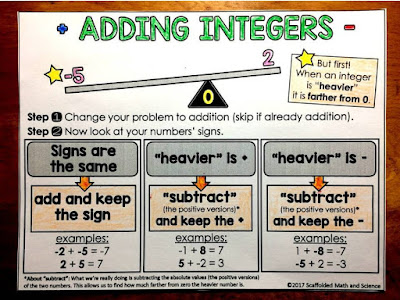Adding and subtracting integers graphic organizer

(You can download this integers graphic organizer for free in this post.)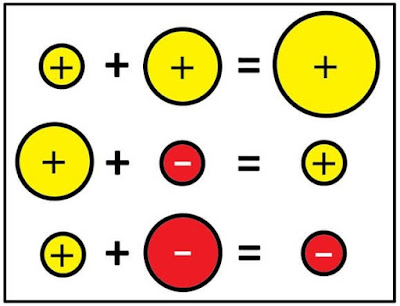Integer rules visuals

I reached out to math teacher and blogger Don Steward about creating some printables based on the visual integers references he had created. Don said that he'd be happy for this to happen. You can find the above poster (plus 3 others covering addition and subtraction) free in this visual integer rules post. There is also a fun graphic for multiplying and dividing integers in the post.

When I taught mainstream Algebra 2, even my strongest students would make mistakes with integer operations. They'd get to the end of solving a complicated radical equation and get tripped up on x - 7 = -3.

10?
-10?
4?
-4?
11?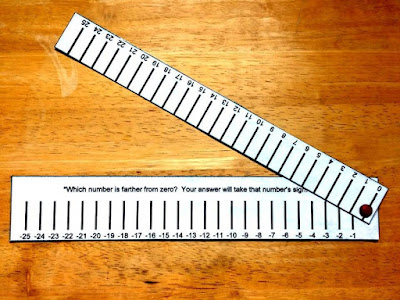Integer Operations Manipulative

When it came time to write my thesis, I wrote it on integers and the integer operations manipulative I created to help make integer operations more concrete.Vertical number line

Linking integers to temperature with a vertical number line may also help some kids internalize these abstract numbers.Divisibility Rules Pennant (in free resource library)

Getting back to numbers as building blocks... I made this divisibility rules pennant (free in math resource library) for students to reference as they are reducing fractions, factoring numbers, finding square roots, etc.Divisibility Rules Pennant (in free resource library)

I posted a list of prime numbers and a list of square numbers on my classroom math word wall. We also have a divisibility rules poster with the same rules as this pennant.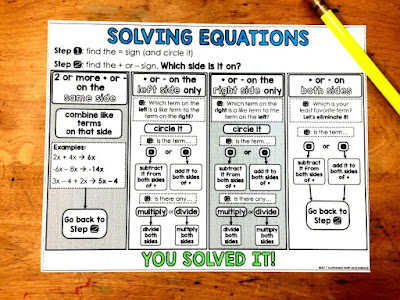Solving equations graphic organizer

Another area where my students have struggled is with solving equations. I made them this solving equations graphic organizer (free) that focuses on the + and - signs as it guides students through the solving process.

It was amazing to me how fast my students were able to solve equations when 30 minutes earlier they didn't even know where to start.

Over the years, this post has become a hodgepodge of ides for teaching number sense. In late 2021, I made a set of multiplication flashcards with strategies for my daughter to help her learn her multiplication facts. You can grab the multiplication flash cards free here.Solving equations using algebra tiles (free solving mat)

A cool way to build number sense in algebra is with algebra tiles. Here is a way to show solving equations using algebra tiles. There are 3 examples with lots of photos, and a link to this free solving mat, in the post.Number classification on an 8th Grade Math Word Wall

I recently added this number classification reference to my 8th grade math word wall to help students visualize different types of numbers.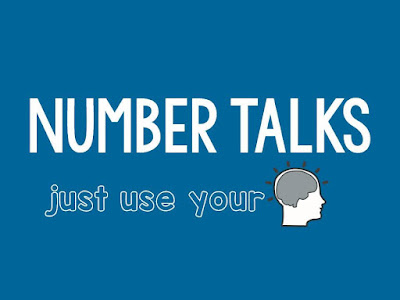Number Talks slides

I had been hesitant for years to give number talks a try in my classes, mainly because I wasn't 100% sure how they worked. When I finally sat down to figure them out and bring them to my class, I wished I hadn't waited so long.

In my consumer math class we worked with percents for our number talks. There is a free set of slides to bring percents number talks to your class in this post.Estimating square roots using visuals

Estimating square can be shown with number lines or with manipulatives to make the topic more concrete. In this video, you can see how we can estimate square roots using visual models. I used cut paper to show the approximations.

With my daughter, we have begun asking her to estimate the number of My Little Ponies she sees in a box or how many crayons are on the table. She has also shown a lot of interest in the Even and Odd Word Wall, building rectangles (even) and jagged shapes (odd) with the pieces that she takes off the wall.

Our hope is to get her number sense ready for later when there will be a time crunch to perform so that her school years may be as happy as ours were years ago.

Here are a few more resources for teaching Number Sense:

Jo Boaler's What is Number Sense video

Don Steward's Directed Number Arithmetic Sped Up

The 5 tips in this post from Top Notch Teaching

10 questions in: How many piano tuners are in NYC?

Blog post: Number Talks in High School Math

Blog post: Integer Rules Visual References

1.1.